One cool thing with Excel is you are able to show values as the Index in Pivot Table. In a nutshell, the Index will tell you the relative importance of a cell i.e. it will show you the relative importance of each value when compared to its row, column, and grand total.

It can help you make decisions if, for example, you want to increase the price of your product, then you will be able to identify the area that will be most impacted (based on the Index).

Don’t forget to download the Exercise Workbook below and follow the step-by-step tutorial on Index in Pivot Table along with us:

STEP 1: Here is our Pivot Table. The two tables are exactly the same.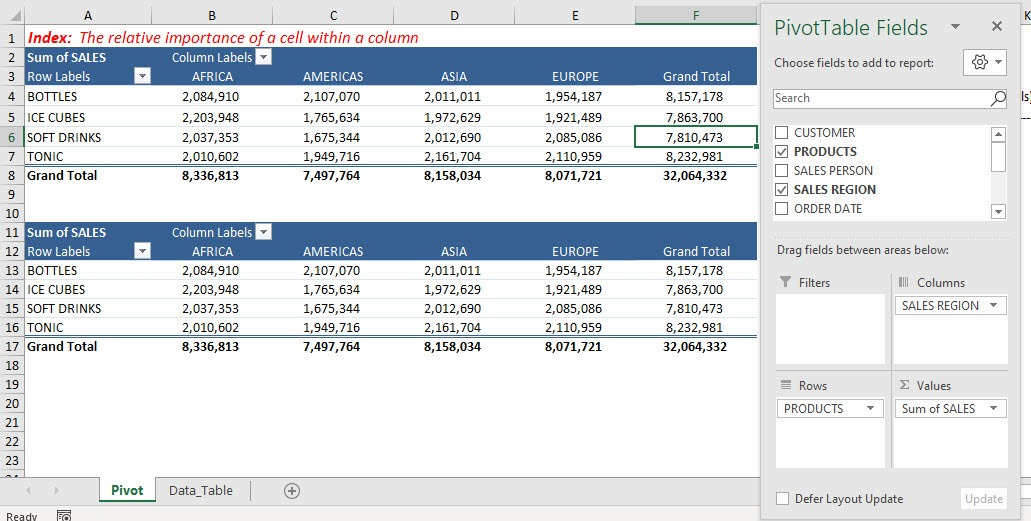STEP 2: Go to the second Pivot Table, and click on the arrow of Sum of SALES and select Value Field Settings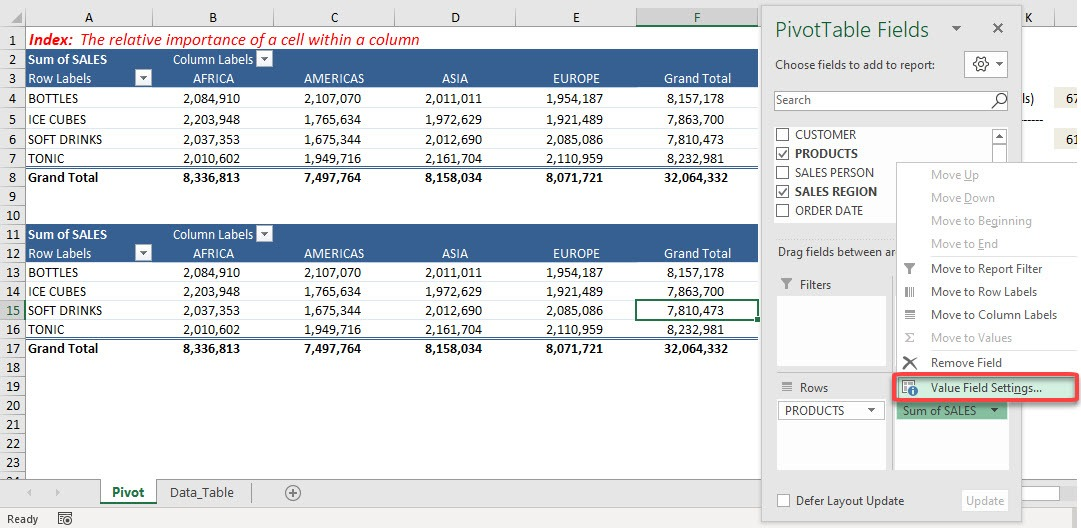STEP 3: Select Show values as > Index. Click OK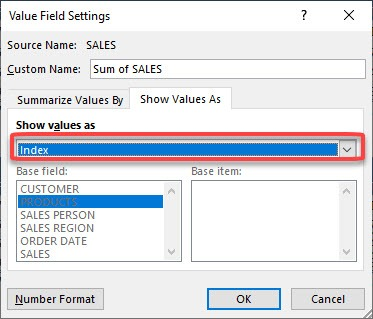STEP 4: Now we have our Index values! The higher value means that it is the most important value for that column, for example, Bottles have the greatest impact on the Americas Region in terms of sales.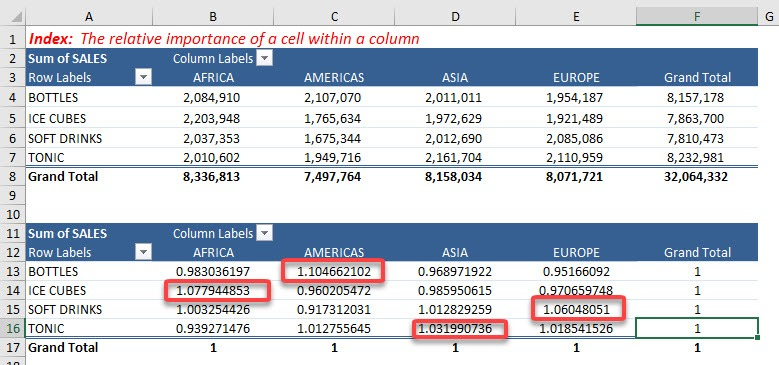Now let’s have a quick calculation to show how Index is calculated. Let us target the Bottles Sales for the Americas Region.

STEP 5: Select the Sales for Bottles-Americas then multiply it by the Grand Total.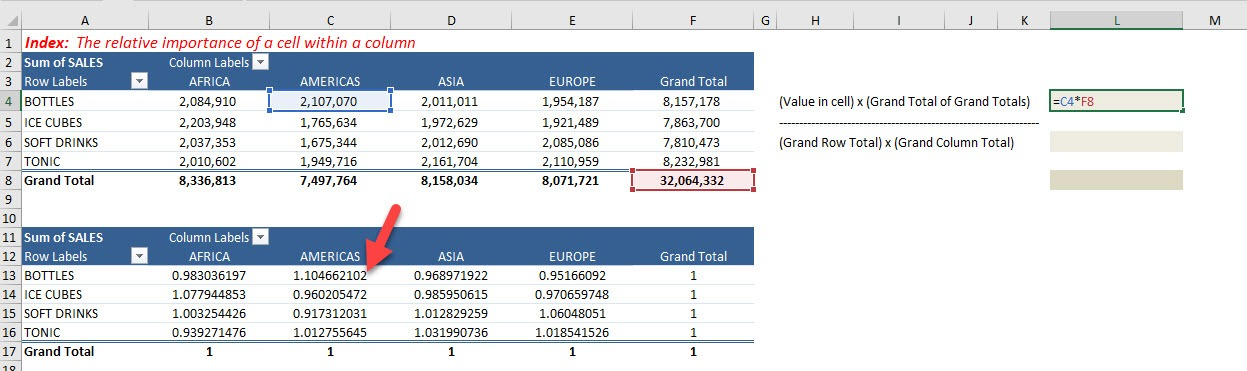STEP 6: Multiply the Grand Row Total of Bottles and the Grand Column Total of Americas.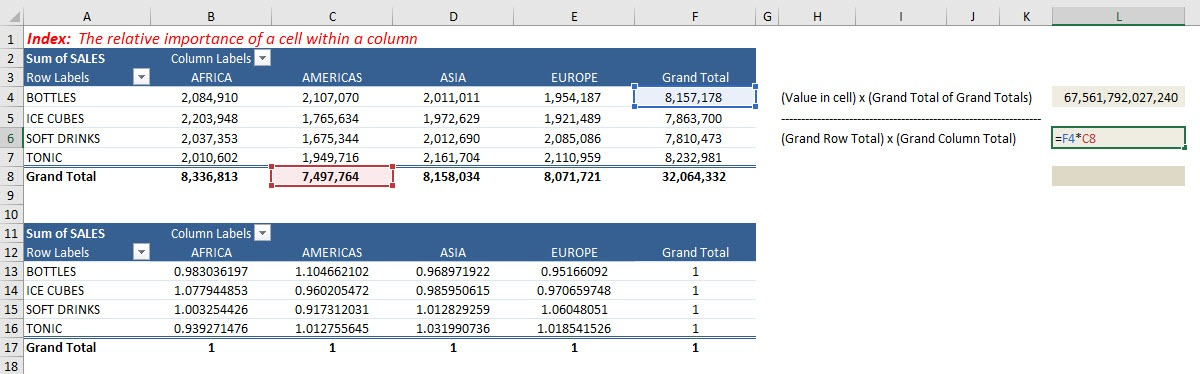STEP 7: Divide them and you will get the Index!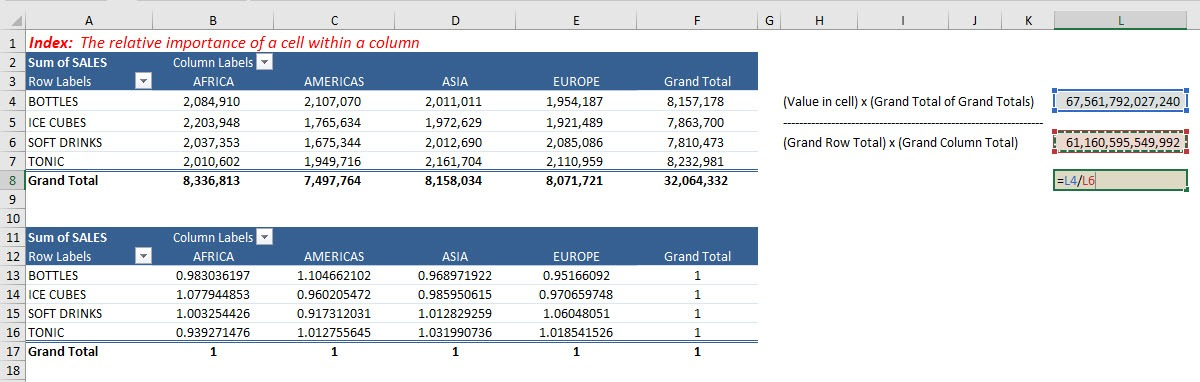The values are exactly the same!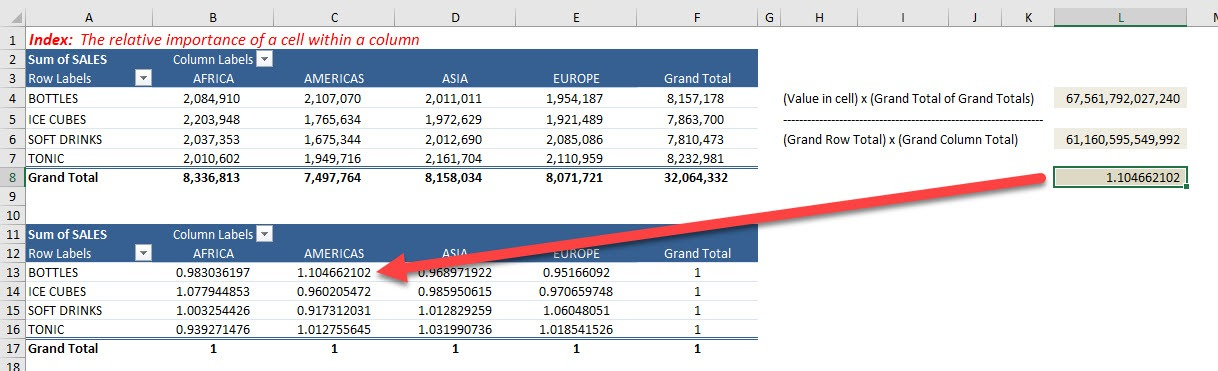Thus, you can calculate the index number for any value by using the formula below:

= (Value of cell * Grand total of Grand Totals) / (Grand Row Total * Grand Column Total)

In this example, you can see that the sales amount of bottles in Africa (2,084,910) and soft drinks in Europe (2,085,086) are almost equal.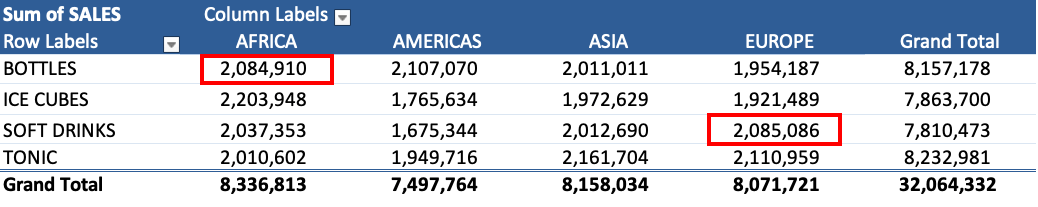But, the index numbers are 0.98 and 1.06.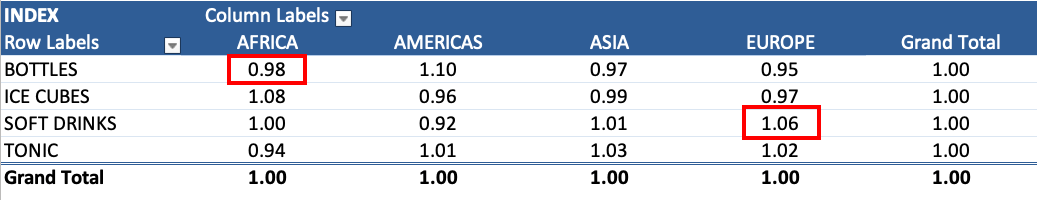The reason behind this difference is that grand total of Africa is greater than that of Europe. So, when the Africa bottles sales amount is divided by a larger number, it results in a lower index number.

These index numbers give the user a clear picture of the importance of each sales amount as per the row, column, and grand totals. The following should be noted with respect to the index number:

• If all the values in the Pivot Table are equal, the index in Pivot Table will be 1.
• If the index in Pivot Table is less than 1, it means that the value is of lower importance than its row and column values.
• If the index in Pivot Table is greater than 1, it means that the value is of greater importance than its row and column values.

There are many more custom calculations in the Pivot Table such as % of Column, % of Row, etc, Click Here to know all about it!